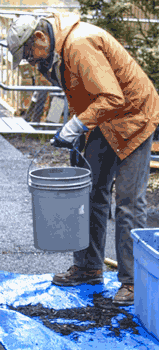Washington State University - Puyallup Organic Farming Systems and Nutrient Management

# Calculating Compost Bulk Density

## Procedure for calculating compost bulk density

1. With a 1-gallon measuring container, fill a 5-gallon bucket with 5 gallons of water, as the top brim of a “5 gallon bucket” is often more than 5 gallons. Mark this line on at least three places on the inside of the bucket with a permanent pen.
1. Buckets with straight sides (ie diameter at the top of the bucket is the same as the diameter at the bottom): measure height from bottom of inside of bucket to the 5 gallon line, divide this number by 3, and mark the inside of the bucket as above at 1× and 2× this number. For example, if the 5-gallon mark is 13 inches, dividing by 3 will get 4.33 inches, so mark that bucket at 4.33 inches and 8.67 inches.
2. If you cannot obtain a bucket with straight sides: find the two intermediate lines by measuring 1 ⅔ gallons (=1.67 gal or 6.31 liters or 1 gallon plus 10 ⅔ cups) then 3 ⅓ gallons (=3.33 gallons or 12.62 liters or 3 gallons plus 5 ⅓ cups) of water into the bucket and marking the inside of the bucket where the water line is at both volumes with a pencil when wet, then a permanent pen when dry.
2. Compost used should be representative of the pile. Take handful samples (not large shovel full samples) from several locations in the pile. Dig into pile a couple of feet, do not take from dried-out outer layer of pile unless the pile was just turned.
3.Fill bucket to the ⅓ line with compost. Drop bucket squarely from approximately 1 foot high to the ground (hard surface) 10 times. Use gravity to drop the bucket, not force.
4. Fill bucket to the ⅔ line. Drop bucket squarely from approximately 1 foot high to the ground 10 times.
5. Fill bucket to the ³⁄₃ (5 gallon) line. Drop bucket squarely from approximately 1 foot high to the ground 10 times.
6. Fill bucket again to the ³⁄₃ (5 gallon) line (do NOT drop bucket!) and weigh in pounds.
7. Multiply weight of material in pounds (excluding bucket weight) by 40. This is your bulk density, in pounds per cubic yard (lbs/yd³).

## Materials Needed

1. Compost (or feedstock) pile
2. 5-gallon bucket (straight sides preferred)
3. Permanent pen marker
4. Measuring containers (gallon, cup, liter)
5. Scale (pounds)
6. Ruler